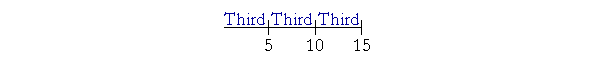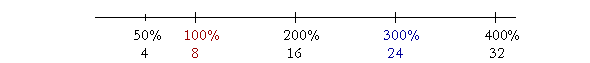S k i l l
i n
A R I T H M E T I C

Lesson 16

# RATIO AND PROPORTION  1

A ratio is a relationship with respect to relative size between two quantities of the same kind: A number of people to a number of people; an amount of money to an amount of money; a distance to a distance.

15 people, for example, are more than 5 people. We can express that by saying how much more, that is, 10 more, or by saying how many times more: Three times more. When we say that 15 people are three times more than 5 people, that is called their ratio.

(For a discussion of saying "times more" versus just saying "times," see below.)

This Lesson depends on Lesson 14: Parts of Natural Numbers

In this Lesson, we will answer the following:

1. What do we mean by the ratio of two natural numbers?
2. How do percents express ratios?

Section 2

3. What is a proportion?

Section 3

4. How can we express the ratio of any smaller number to a larger number?
5. When is a ratio expressed with the lowest terms?
6. How can we know when a ratio is expressed with the lowest terms?
7. What is the Theorem of the Common Divisor?

 1. What do we mean by the ratio of two natural numbers? It is their relationship with respect to relative size which we can express in words. Specifically, one number is a multiple of the other (so many times it), a part of it, or parts of it.

(Euclid, Book VII. Def.20.)

Example 1.  Multiple.   What ratio has 15 to 5?

Answer.  15 is three times 5.

That is the ratio -- the relationship -- of 15 to 5.  If Jill has \$15, and Jack has \$5, then Jill has three times more than Jack.

We do not answer "3 to 1," because we want to name the ratio of 15 to 5 explicitly. It is true that 15 is to 5 as 3 is to 1 -- but what ratio has 3 to 1?  3 is three times 1.

(The 19th century program to rid mathematics of language and replace it with algebraic relations, successfully put to sleep the subject of ratio and proportion.)

Notice that we answer with a complete sentence beginning with the first number 15 and ending with the last number 5.  For, a ratio is a relationship.

The two numbers in a ratio are called the terms; the first and the second.

When the first term is larger, we say it is so many times the smaller number.  15 is three times 5.

What ratio has 28 to 7?

28 is four times 7.

Example 2.  Part.   What ratio has 5 to 15?

Answer.  5 is the third part of 15.

That is called the inverse ratio of 15 to 5. The terms are exchanged.

Notice again that we answer with a complete sentence beginning with the first term and ending with the second.  "5 is              15."

Example 3.  Parts.   What ratio has 10 to 15?Answer.  10 is two thirds of 15.

These are the three types of ratio.  One number is a multiple of the other (so many times it), a part of it, or parts of it.

Example 4.   What ratio has 12 to 6?

Answer.  12 is two times 6. Or we could say, "12 is twice as much as 6," or "12 is double 6."

These are the various ways of expressing the ratio, the relationship, of 12 to 6.

Inversely, 6 is half of 12.

When trying to express a ratio, if the student will say a sentence, and then consider the truth of that sentence, the fact will speak for itself.

Example 5.   What ratio has 6 to 30?  And inversely, what ratio has 30 to 6?

Answer.  6 is the fifth part of 30.  Inversely, 30 is five times 6.

Example 6.   What ratio has 63 to 7? And inversely, what ratio has 7 to 63?

Answer.  63 is nine times 7.  Inversely, 7 is the ninth part of 63.

 2. How do percents express ratios? Whatever ratio the percent has to 100 percent, that is the ratio we mean.

Example 7.   What ratio has 200 to 100?

Answer.  200 is two times 100, or twice as much as 100.

200%, then, means twice as much.  200% of 6 -- two times 6 -- is 12.

Example 8.   How much is 300% of 8?

Answer.  24.  300% of 8 means three times 8, because 300 is three times 100.100% is the whole, in this case, 8.  50% means half, because 50 is half of 100.  50% of 8 is 4.

200% means two times, because 200 is two times 100.  200% of 8 is 16.

300% means three times; 400% means four times; and so on.

Whatever ratio the percent has to 100 percent, that is the ratio we mean.

What is more, we see that any number less than 8 will be less than 100% of 8.  While any number more than 8 will be more than 100%.

We have, as it were, two languages:  The language of ratio -- "Half,"  "Three quarters," "Twice as much" -- and the language of percent:  50%, 75%, 200%.  The student must become fluent in both languages, and in translating from one to the other.

Example 9.   Compare the following:

a)  10 has what ratio to 40?     b)  10 is what percent of 40?

Answer.  a)  10 is the fourth part of 40; or a quarter of 40, or a fourth of 40.

b)  10 is 25% of 40.

25% means a quarter, because 25 is a quarter of 100.

*

15 is more than 5. How many times more?

15 is three times more. No? Yet some critics insist that we should not say, "15 is three times more than 5" -- because they say that should mean 20. Why? Because three times 5 is 15. So three times more than 5 should mean 5 + 15, which is 20.

There is good reason to reject that argument, however, and it is simply because of our normal and idiomatic spoken language. There is nothing the least ambiguous about saying, "15 is three times more than 5."

As an example of consistency, these critics cite, "How much is 50% more than 10?" To that question it is correct to answer 15. But that is simply another idiomatic use of language when speaking of percent. Though it might seem to follow logically that we should speak that way in all cases, it does not follow linguistically. Mathematicians cannot require us to speak a private language.

As Merriam-Webster's Dictionary of English Usage puts it:

"The question to be asked ... is not whether it makes sense mathematically, but whether it makes sense linguistically — that is, whether people understand what it means. ... The 'ambiguity' of times more is imaginary: in the world of actual speech and writing, the meaning of times more is clear and unequivocal. It is an idiom that has existed in our language for more than four centuries, and there is no real reason to avoid its use."

So, 15 is more than 5. No? How many times more?

15 is three times more than 5.

or

Continue on to the next Section:  Proportions

1st Lesson on Parts of Natural Numbers

www.proyectosalonhogar.com